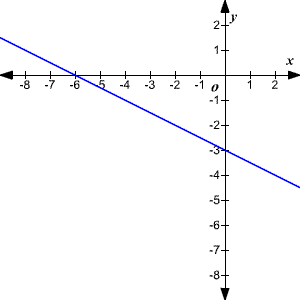# Finding the Equation of a Line from Its Graph

Suppose you are given the graph of a line in the coordinate plane, and asked to find its equation.

If you can find the $y$ -intercept and the slope , you can write the equation in slope-intercept form (unless, of course, it's a vertical line .)

Example :

Find the equation of the line shown.Here the line crosses the $y$ -axis at $-3$ , so the $y$ -intercept is $-3$ .

To find the slope, use the two points $\left(-6,0\right)$ and $\left(0,-3\right)$ and substitute these in the slope formula:

$\begin{array}{l}m=\frac{{y}_{2}-{y}_{1}}{{x}_{2}-{x}_{1}}\\ \text{\hspace{0.17em}}\text{\hspace{0.17em}}\text{\hspace{0.17em}}\text{\hspace{0.17em}}\text{\hspace{0.17em}}=\frac{-3-0}{0-\left(-6\right)}\\ \text{\hspace{0.17em}}\text{\hspace{0.17em}}\text{\hspace{0.17em}}\text{\hspace{0.17em}}\text{\hspace{0.17em}}=-\frac{1}{2}\end{array}$